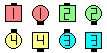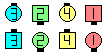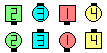Definitions of Square Dance Calls and Concepts
Bias Circulate [C3A]Index -->  Plus  |  A1  |  A2  |  C1  |  C2  |  C3A  |  C3B  |  C4  |  NOL  |
Definitions (Text Only) -->  Plus  |  A1  |  A2  |  C1  |  C2  |  C3A  |  C3B  |  C4  |  NOL  |
 Find call:

 \$B8@8l(B\$BJ?9T\$J(B Wave \$B\$^\$?\$OB>\$NE,@Z\$J(B formation \$B\$+\$i!%(B

Leaders \$B\$O(B Circulate \$B\$r(B, Trailers \$B\$O(B Diagonal Box Circulate \$B\$r\$7\$^\$9!%(B \$BDL\$jJ}(B: \$B1&\$N(B Wave \$B\$+\$i\$O(B Trailers \$B\$O1&

\$BJ?9T\$J(B Wave \$B\$+\$i\$O(B, \$BJ?9T\$J(B Wave \$B\$G=*\$o\$j\$^\$9!%(BBias Circulate\$B\$NA0(B \$B8e(B

\$BCm

• For Trailers: Ends become Centers, and Centers become Ends.
• C3B definition: Leaders Circulate as Trailers Diagonal Box Circulate.
• The original definition of Bias Circulate had the Trailers make a R-H (or L-H) Star, Turn it 1/2 way, and adjust to end in Waves. Hence, it was possible for the caller to modify the amount to turn the Star (this is considered bogus today). Our definition uses Diagonal Box Circulate as a convenient way to express turning the Star. It is inappropriate to call Bias Circulate from T-Bones such that the Trailers are in Columns instead of Lines.
• Bias Circulate \$B\$O(B, Trapezoid Split Trade Circulate \$B\$HF1\$87k2L\$K\$J\$j\$^\$9(B (\$B:8\$N(B Wave \$B\$+\$i\$O:8\$N(B Star \$B\$r:n\$k\$H\$\$\$&\$3\$H\$r=|\$\$\$F(B)\$B!%(B
• Bias Trade Circulate [C4]: \$BJ?9T\$J(B Wave \$B\$+\$i!%(B \$B0l\$D\$NF0\$-\$H\$7\$F(B, Bias Circulate and Spread \$B\$r\$7\$^\$9!%(B \$B5U\$NBias Trade Circulate\$B\$NA0(B \$B8e(B

\$BDiagonal Box CONCEPT [C3B].CALLERLAB definition for Bias CirculateChoreography for Bias CirculateComments? Questions? Suggestions?